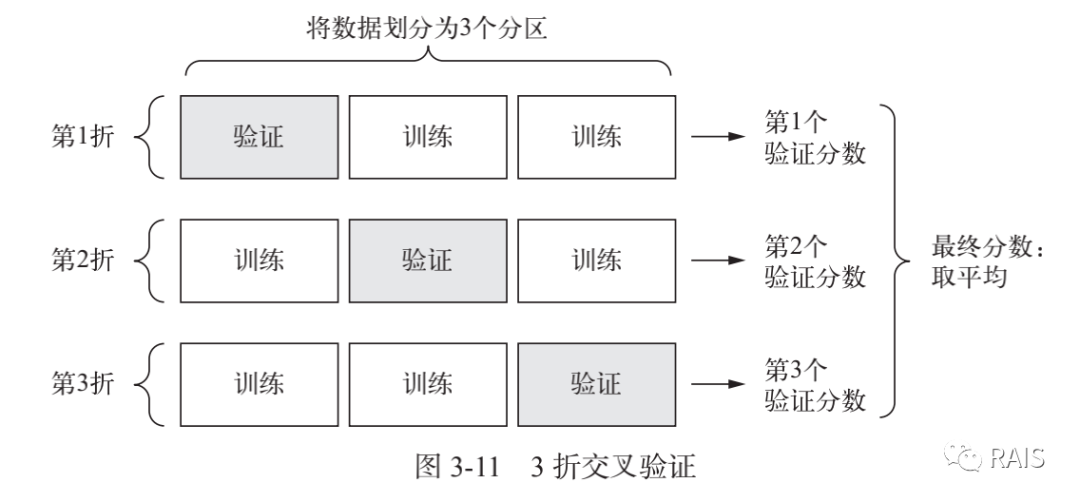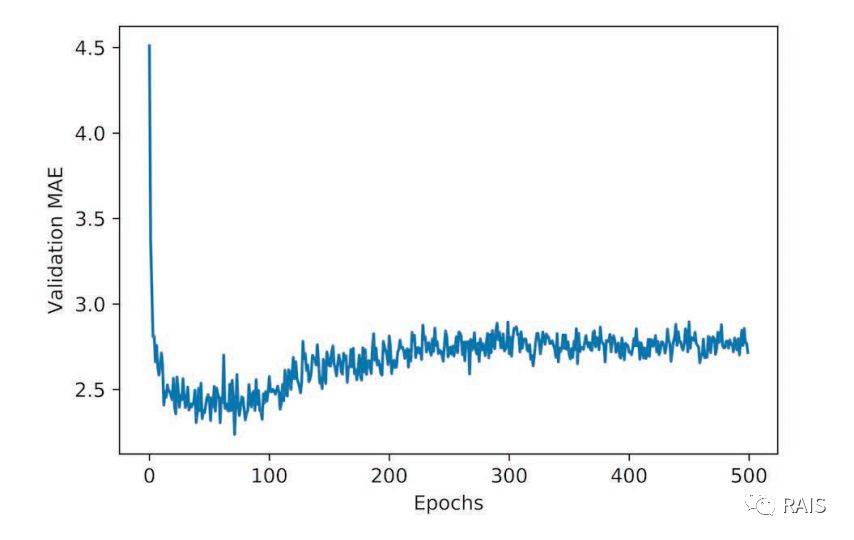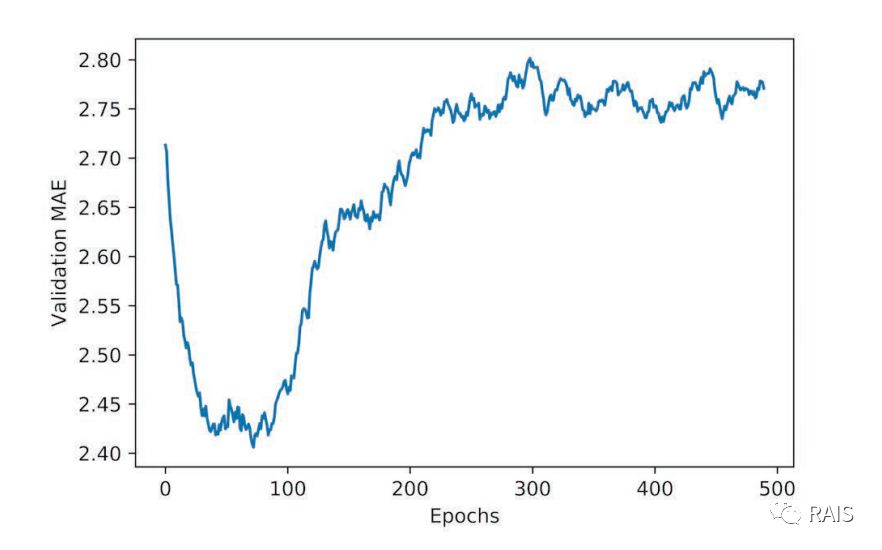# 预测房价：人工智能回归问题

2019/04/10 10:10

​我们之前提出了三个经典的问题，他们分别是：

1. 二分类问题（电影评论好坏倾向性判断）
2. 多分类问题（将新闻按照主题分类）
3. 回归问题（根据房地产数据估算房地产价格）

1. 从数据集中读取数据我们已经很熟悉了，但是我们观察数据会发现，这些数据的取值范围差别太大了，这会导致网络训练过程的失真，因此比较好的办法是我们先对数据进行预处理，预处理的方法是：(原数据 - 平均值) / 标准差，这就相当于对数据进行标准化，标准化后的数据平均值为 0，标准差为 1。mean 和 std 方法分别是求平均值和计算标准差。

2. 因为我们这一次的数据量只有五百多个，因此我们采用较小的网络，两个隐藏层。这里我们需要注意的一点是数据量少，训练容易产生过拟合，小型网络更适合。

3. 我们仍然可以用之前的方法进行训练集与反馈集的划分，但问题是由于我们的数据量太小了，因此具体如何划分反馈集过于随机，这会对最后的结果有很大的影响，因此我们采用的是 K 折交叉验证的方法。K 折交叉验证的含义是我们将数据集分为 K 份，每次从这 K 份中选择一份当做验证集，进行 K 次互相独立的训练，最后取 K 次训练的平均值。具体如图：4. 我们画出训练 500 轮的图，可以看到最开始的一些数据不是好数据，我们把他们去掉，然后再绘制一张图，如下别是两次绘制的结果，又可以看到之前出现的问题——过拟合了，因此我们调整循环次数为 80 次5. 修改后的训练网络是一个可以接受的网络，我们在测试集上进行验证，整体基本可以达到要求。

• 神经网络对数据的处理大多都需要转化为对数字的处理，因此对于文本等内容需要进行预处理；

• 对于数据集的大小、特征的多少和特征值之间的差别等，考虑数据网络的大小，层数、数据的标准化和训练的迭代次数，此类问题往往也需要画图去观察和判断，最后需要根据调整的参数最终得到比较合适的网络模型；

• 训练迭代次数不够和过拟合都是经常遇到的问题，都是不够好的训练网络，实际问题中需要对两种情况都进行评估和调整；

• 损失函数和反馈函数的选取，需要考虑实际问题，根据数据的要求，进行选择；

#!/usr/bin/env python3

import time

import numpy as np
from keras import layers
from keras import models
from keras.datasets import boston_housing

def housing():
global train_data

(train_data, train_targets), (test_data, test_targets) = boston_housing.load_data()
# (404, 13)
# print(train_data.shape)
# (102, 13)
# print(test_data.shape)
# [15.2 42.3 50.  21.1 17.7 18.5 11.3 ... 19.4 19.4 29.1]
# print(train_targets)

# 平均值
mean = train_data.mean(axis=0)
train_data -= mean
# 标准差
std = train_data.std(axis=0)
train_data /= std
test_data -= mean
test_data /= std

k = 4
num_val_samples = len(train_data) // k
num_epochs = 500
all_mae_histories = []
for i in range(k):
print('processing fold #', i)
val_data = train_data[i * num_val_samples: (i + 1) * num_val_samples]
val_targets = train_targets[i * num_val_samples: (i + 1) * num_val_samples]
partial_train_data = np.concatenate(
[train_data[:i * num_val_samples],
train_data[(i + 1) * num_val_samples:]],
axis=0)
partial_train_targets = np.concatenate(
[train_targets[:i * num_val_samples],
train_targets[(i + 1) * num_val_samples:]],
axis=0)
model = build_model()
model.fit(train_data, train_targets,
epochs=80, batch_size=16, verbose=0)
test_mse_score, test_mae_score = model.evaluate(test_data, test_targets)
# history = model.fit(partial_train_data, partial_train_targets,
#                     validation_data=(val_data, val_targets),
#                     epochs=num_epochs, batch_size=1, verbose=0)
# mae_history = history.history['val_mean_absolute_error']
# all_mae_histories.append(mae_history)

# average_mae_history = [
#     np.mean([x[i] for x in all_mae_histories]) for i in range(num_epochs)]
#
# plt.plot(range(1, len(average_mae_history) + 1), average_mae_history)
# plt.xlabel('Epochs')
# plt.ylabel('Validation MAE')
# plt.show()
#
# smooth_mae_history = smooth_curve(average_mae_history[10:])
# plt.plot(range(1, len(smooth_mae_history) + 1), smooth_mae_history)
# plt.xlabel('Epochs')
# plt.ylabel('Validation MAE')
# plt.show()

def build_model():
model = models.Sequential()
input_shape=(train_data.shape,)))
model.compile(optimizer='rmsprop', loss='mse', metrics=['mae'])
return model

def smooth_curve(points, factor=0.9):
smoothed_points = []
for point in points:
if smoothed_points:
previous = smoothed_points[-1]
smoothed_points.append(previous * factor + point * (1 - factor))
else:
smoothed_points.append(point)
return smoothed_points

def smooth_curve(points, factor=0.9):
smoothed_points = []
for point in points:
if smoothed_points:
previous = smoothed_points[-1]
smoothed_points.append(previous * factor + point * (1 - factor))
else:
smoothed_points.append(point)
return smoothed_points

if __name__ == "__main__":
time_start = time.time()
housing()
time_end = time.time()
print('Time Used: ', time_end - time_start)


0
0 收藏

### 作者的其它热门文章0 评论
0 收藏
0